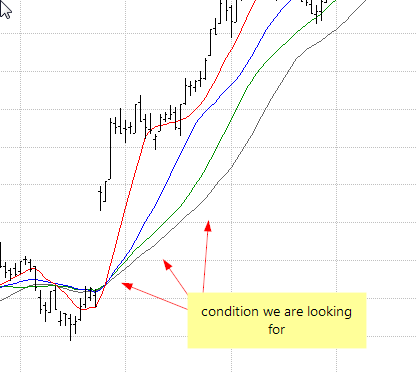amibroker

Checking relationship between multiple moving averages

When we compare the positions of several lines against one another, we need to remember about using correct operators, so our AFL statement return correct results. Let us consider a set of moving averages using 10, 20, 30 and 40 periods, drawn with the following code:

MA10 MAClose10 );
MA20 MAClose20 );
MA30 MAClose30 );
MA40 MAClose40 );

PlotClose"Close"colorDefaultstyleBar );
PlotMA10"MA10"colorRed );
PlotMA20"MA10"colorBlue );
PlotMA30"MA10"colorGreen );
PlotMA40"MA10"colorgrey40 )When we want to specify a condition where MA10 is highest of all of the lines, above MA20, which is above MA30 and with MA40 on the very bottom – we can NOT simply write:

condition MA10 MA20 MA30 MA40// WRONG - NOT what we really want, but no syntax erro

It may seem strange that such statement is accepted without an error but it is actually syntactically correct. This is because of the fact that True and False are represented by numbers 1 and 0 respectively, so all comparisons actually have numerical value that allows such statement to be evaluated and yield numeric result. The above statement is evaluated from left to right and would be an equivalent of:

condition = ( ( MA10 MA20 ) > MA30 ) > MA40// again WRON

Using > operator will return an array of True or False values (1 or 0). Therefore – the result of MA10 > MA20 comparison (which is True, that is equal to 1) would be then compared to MA30, resulting in checking 1 > MA30, then if such condition returns False (i.e. 0 ), we would end up with 0 > MA40 comparison that would return False (0) as the final output. This is of course not what we want to get.

That is why we should use AND operator instead, because we want to check several conditions being met at the same time, that is:

MA10 is above MA20
AND // must use AND/OR to combine multiple conditions
MA20 is above MA30
AND // must use AND/OR to combine multiple conditions
MA30 is above MA40

Therefore, we should write the AFL statement the following way:

condition MA10 MA20 AND MA20 MA30 AND MA30 MA40// correct way of checking multiple condition

So as a general guideline – if you have multiple boolean (yes/no) conditions that you want to combine into single rule, you need to use AND operator between conditions if you want True result when all conditions are met at the same time.

In a similar way, if you want a True result when any one (or more) of multiple conditions is met, then you need to use OR operator.

condition MA10 MA20 OR MA20 MA30// this will give True result if any one condition (or both) is me